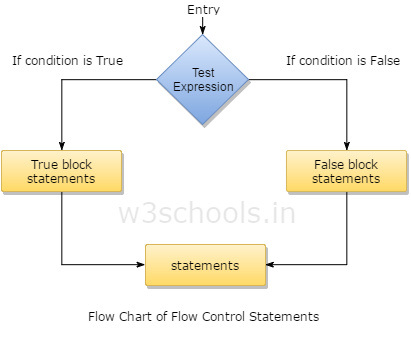##### R Programming Tutorial Index

In R programming like that with other languages, there are several cases where you might wish for conditionally execute any code. Here 'if' and 'switch' functions of R language can be implemented if you already programmed condition based code in other languages, Vectorized conditional implementation via the ifelse() function is also a characteristics of R. In this chapter you will look at all of these conditional statements that R provides programmers to write.

## What is Flow Control in R?

There are a lot of situations where you do not just want to execute one statement after another: in fact you have to control the flow of execution also. Usually this means that you merely want to execute some code if a condition is fulfilled. In that case control flow statements are implemented within R Program.Here is the general structure of how control flow can be handled using the conditional statements within R programming.

## Types of Flow Control Statements in R Programming

R programming provides three different types if statements that allows programmers to control their statements within source code. These are:

• if statement
• if….else statement
• switch statement

### The 'if' Statement

The simplest form of decision controlling statement for conditional execution is the 'if' statement. The 'if' produces a logical value (more exactly, a logical vector having length one) and carries out the next statement only when that value becomes TRUE. In other words, an 'if' statement is having a Boolean expression followed by single or multiple statements.

``````if (TRUE)        print ("One line executed")
## One line executed
if (FALSE)       print ("Line not executed")
## Line not executed
if (NA)  print ("Don't know whether true or not!")
## Error: missing value where TRUE/FALSE needed
``````

### The 'if….else' statements

In this type of statements the 'if' statement is usually followed by an optional 'else' statement that gets executes when the Boolean expression becomes false. This statement is used when you will be having multiple statements with multiple conditions to be executed.

Example:
``````if (TRUE)
{
print ("This will execute...")
} else
{
print ("but this will not.")
}
## This will execute...
``````

### The 'switch' Statement in R

A switch statement permits a variable to be tested in favor of equality against a list of case values. In the switch statement, for each case the variable which is being switched is checked. This statement is generally used for multiple selection of condition based statement.

The basic syntax for programming a switch based conditional statements in R is:
Syntax:

``switch (test_expression, case1, case2, case3 .... caseN)``

Here is a simple example of how to make use of switch statement in R:

Example:
``````gk  <-  switch (
2,
"First",
"Second",
"Third",
"Fourth"
)
print (gk)
##  "Second"``````